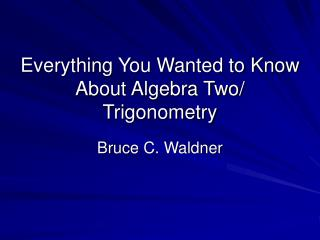Download PresentationEverything You Wanted to Know About Algebra Two/ Trigonometry

Loading in 2 Seconds...

# Everything You Wanted to Know About Algebra Two/ Trigonometry - PowerPoint PPT Presentation

Everything You Wanted to Know About Algebra Two/ Trigonometry Bruce C. Waldner Contact Information bcwaldner@aol.com Coordinator of Mathematics, K – 12 Syosset Central School District Syosset High School 70 SouthWoods Road Syosset, New York 11754I am the owner, or an agent authorized to act on behalf of the owner, of the copyrighted work described.
Download Presentation## Everything You Wanted to Know About Algebra Two/ Trigonometry

An Image/Link below is provided (as is) to download presentation

Download Policy: Content on the Website is provided to you AS IS for your information and personal use and may not be sold / licensed / shared on other websites without getting consent from its author.While downloading, if for some reason you are not able to download a presentation, the publisher may have deleted the file from their server.

- - - - - - - - - - - - - - - - - - - - - - - - - - E N D - - - - - - - - - - - - - - - - - - - - - - - - - -
Presentation Transcript
1. Everything You Wanted to Know About Algebra Two/ Trigonometry Bruce C. Waldner

2. Contact Information bcwaldner@aol.com Coordinator of Mathematics, K – 12 Syosset Central School District Syosset High School 70 SouthWoods Road Syosset, New York 11754

3. Changes in the Mathematics Curriculum The new New York State Mathematics Learning Standards

4. Is it really what it sounds like? • Three proposed Regents Examinations • Integrated Algebra • Integrated Geometry • Integrated Algebra 2 and Trigonometry

5. NYS Regents program over the years

6. The new proposed NYS Regents program in Mathematics • Reverts to a more traditional sequence of high school mathematics courses • Includes the real-world connections and the constructed response format of questions found in Math A and Math B • Three Regents examinations • Graphing calculators needed • Includes probability, and statistics as in the Sequential Math program and Math A and Math B as well as some of the logic • More in-sinc with other parts of the country • Based on NCTM Standards

7. The current Regents program Math A – June of 9th grade (grade 8 for accelerated students) Math B – June of 11th grade (grade 10 for accelerated students) The new Regents program Integrated Algebra – June of 9th grade (or grade 8) Geometry – June of 10th grade (or grade 9) Integrated Algebra and Trigonometry – June of 11th grade (or grade 10) When will students take the Mathematics Regents Examination(s)?

8. Normal Approximation • In a binomial distribution of n trials, the mean = np and the standard deviation = or. Let r represent the number of successes in n trials. Since the data in a binomial distribution is discrete rather than continuous, then to estimate the probability of at least r successes in n trials, it is necessary to subtract 0.5 • from r .

9. New topics • The two major new topics are: • Normal Approximation to a Binomial Distribution • Sequences and Series Check out the handout taken from the NYSED Crosswalk for Algebra Two/Trigonometry

10. Normal Approximation for a Binomial Probability Math Facts

11. Example 1 A manufacturer of light bulbs knows that the probability that a light bulb produced by his company being defective is 0.01. Out of 30 light bulbs sold to one of his customers, use a normal distribution to approximate the probability that no more than 3 are defective?

12. manual solution

13. Solution to Example 1 • In this binomial distribution the mean = and the standard deviation = . . The graphing calculator can be used to determine this result using a low value of 0.5 lower than the least possible number 0 or –0.5 and 3.5 as the highest number, mean = 0.3 and standard deviation of 0.545, press • 2nd VARS 2 –0.5 , 2.5 , 0.3 , 0.545 ) ENTER .

14. Calculator screen

15. Example 2 Anytime Gary plays James in a game of chess, he has a 70% probability of winning the game. If they play 10 chess games, use a normal distribution to approximate the probability that Gary wins at least 8 games.

16. Solution to Example 2 • In this binomial distribution the mean = 10(.7) = 7 and the standard deviation = . .

17. Using the calculator the answer is revealed as: